# Algorithms Complexity Evaluation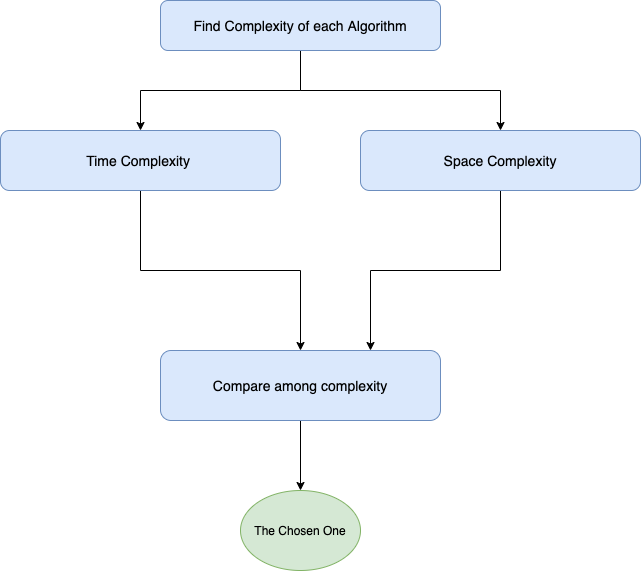Complexity of an algorithm is a measure of the amount of time and/or space required by an algorithm for an input of a given size.

# Basics

• Complexity measure in machine independent manner
• Any algorithm consists of indivisible process operation or step
• Each step take same amount of time to execute
• Algorithms running time measured in processor operations instead of seconds
• This type of measurement is called DTIME or TIME
• DTIME: Number of computation step a computer would take for a solution using certain algorithm

# Time Complexity

Time complexity is a function that represents dependency between input data and time it take to complete all the computations. As we need to find worst case time complexity we need to consider N (towards infinity)as big as possible.

• Rule 1: Always worst Case
• Rule 2: Remove Constants, find the leading term
• Rule 3: Different inputs should have different variables. For consecutive action complexity will be Addition, O(a+b). For nested it would be Multiplications, O(a*b) + for steps in order * for nested steps
• Rule 4: Drop Non-dominant terms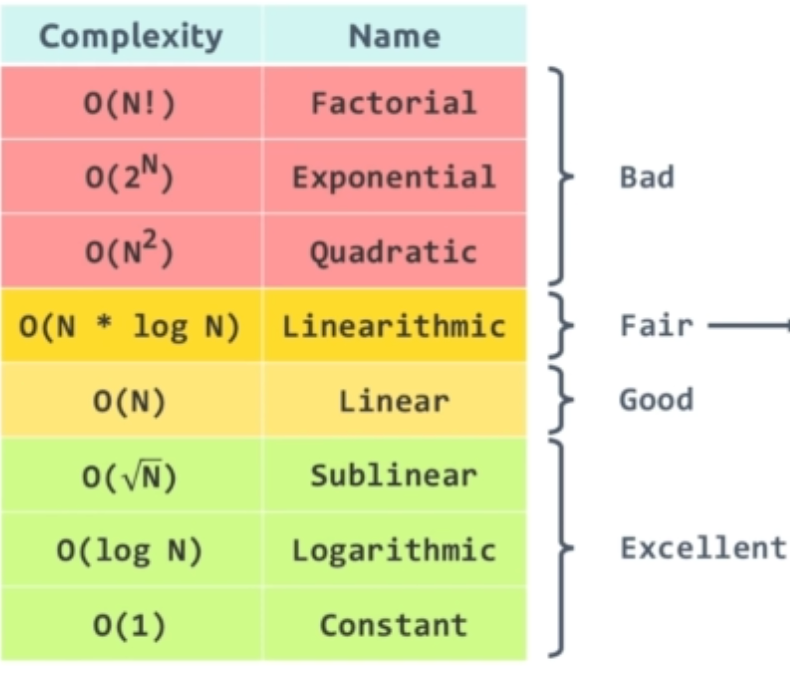Functions sorted by desc order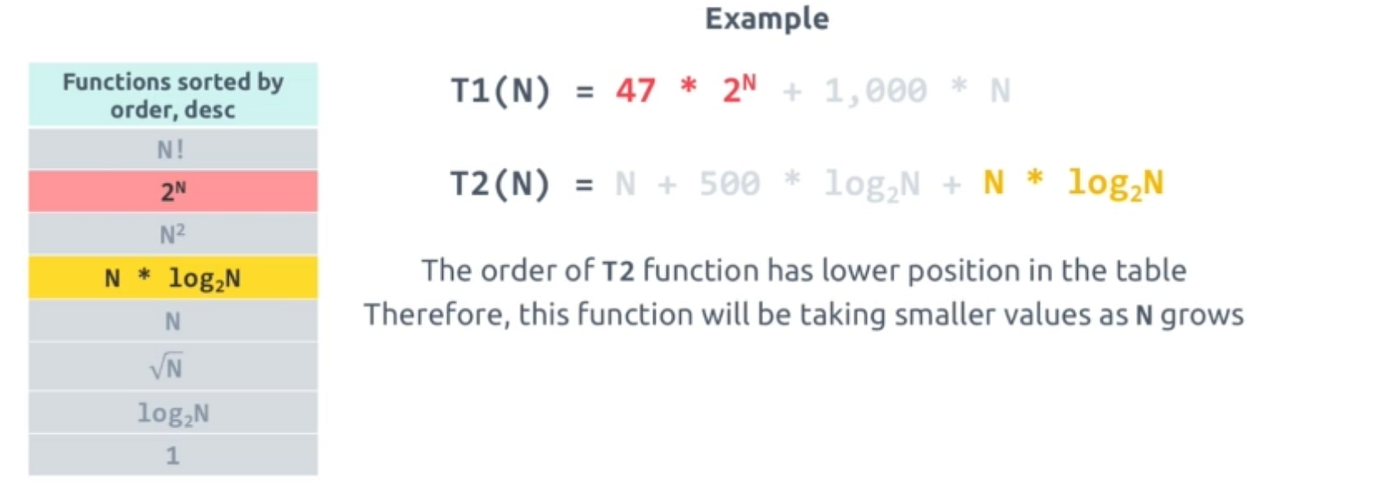An example to depict time comparison between two function

# Big O notation

• Big O notation is used to classify algorithms according to how their run time or space requirements grow as the input size grows.
• The letter O is used because the growth rate of a function is also referred to as the order of the function.
• If f(x) is a sum of several terms, if there is one with largest growth rate, it can be kept, and all others omitted.
• If f(x) is a product of several factors, any constants (terms in the product that do not depend on x) can be omitted.

# Complexity Evaluation

Few useful formulas for complexity evaluation

• 1 + 2 + 3 + 4 + … +N = N²/2 = O(N²)
• 2⁰+2¹+2²+….+ 2^n = *2^N - 1 = O(2^n)
• log a N = log a b+log b N = O(log N) (a, b const)

# Log N complexity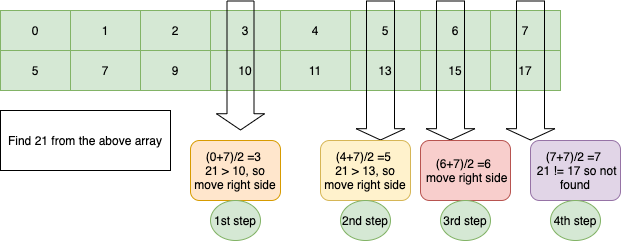Complexity in Binary Search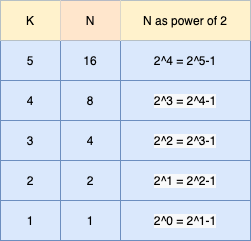Relation between K and N is N = 2^k-1

# String Complexity Evaluation

## 1. Compare String

• Comparing two String to find which one greater string processor need to compare between two arrays of characters.
• In worst case the loop needs to iterate over full length of one array
• So, resulting complexity is O(s1.length) = O(N)

## 2. String Concatenation

• Strings are immutable in Javascript, ruby, Java and many other language
• This mean every string operation will create a new string in memory
• For every string concatenation (“ab” + “cde”) of length N and K will bellow algorithm and complexity will be O(N + K)

## 3. Getting the Substring

Complexity of getting a substring is O(end — start) = O(N-K)

## 4. Recursive Functions Complexity

To evaluate complexity of recursive function
1. Calculate complexity of a single function call
2. Number of recursive call
3. Multiply number of recursive call by single call complexity

`function foo(n){    if(n==1)return 1;        --------> complexity 1    return n+foo(n-1); -->    foo(4) => foo(3) => foo(2) => foo(1)                       --> N number of recursive call   };`
`function foo(n){    if(n==0)return 1;        --------> complexity O(1)    return n+foo(n-1); -->    foo(4) => foo(3) => foo(2) => foo(1)                       --> N number of recursive call   };`
• Above function has one recursive call with step -1
• Chain of call will be from N to 0, => N+1 times
• Thus, complexity is O(1) * (N+1) = O(N)
`Example:function foo(n){    if(n==0)return;    --------> complexity O(1)    foo(n/2)   // N/2 number of recursive call                // when an algorithm process half of the                                                                                                                            elements per iteration, will have long N complexity                            };`
`function fib(n, cache){            // As no operation depends on N    if(n<=1){return n};           // Single call complexity is O(1)    if (cache[n] == undefined){        cache[n] = fib(n-1, cache) + fib(n-2, cache); // Cache the recursive call result    }    return cache[n];                       };function printFib(n){    let cache = [];                         for(i=0; i<=n; i++){        const nthFib = fib(i, cache); // fib function executed fro 0 to N        console.log(nthFib);    }};`
• First 2 calls have no dependency on N so, O(1)
• Starting from N=2, every call finish in 3 calls\
• So total call will be 1 + 1 + 3*(N-1) = 3N -1 = O( N)
• Therefore, complexity of the algorithm will be O(1)*O(N) = O(N)

# Few Interesting Pitfalls

Since Big O Notation tells us about the upper bound of an algorithm ignoring constants and low-order terms. An algorithm may have exact number of steps in the worst case more or less than the Big O notation.

## Exact Steps > Big O

An algorithm takes 5 * N steps has complexity of O(N) in the worst case, which is smaller than the exact number of steps.

`for(i = 0; i < 5*n; i++ )    k++;`

## Exact Steps < Big O

Another example is, when the number of loops are decreasing by the time. So, first time it will loop N, N-1, N-2, …, till 1. The Big O notation for the following code is O(N³), while the exact number of steps is much less than that.

`for (int i = 0; i < n; ++i)    for (int j = i; j < n; ++j)        for (int k = j; k < n; ++k)            cunt++;`

# Space Complexity

Amount of memory required for an algorithm to run in a computer

• Space complexity function is denoted as S
• All Big O notation rule for time complexity holds true for space complexity evaluation too
• For normal function space complexity is O(1)
• For recursive function space complexity S(N) = O(1)*N= O(N)
• For string concatenation O(n²)

--

--